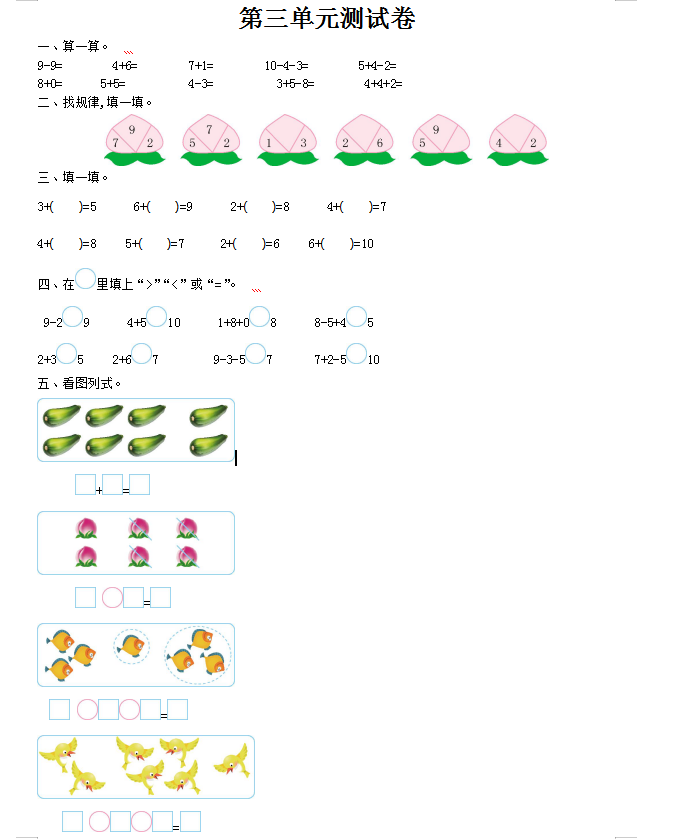9-9=　　　　4+6=　　　　7+1=　　　　10-4-3=　　　　5+4-2=

8+0=              5+5=                4-3=                  3+5-8=                   4+4+2=